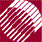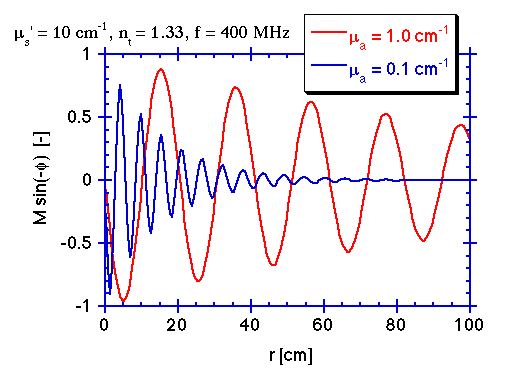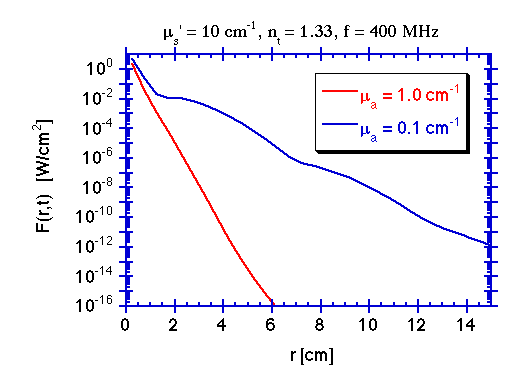ECE532 Biomedical Optics © 1998 Steven L. Jacques, Scott A. Prahl Oregon Graduate Institute

# Frequency-domain Diffusion theory

## Examples

The following figure illustrates the time-resolved signal F(r,t) in blue and the source S(t) in red. Note the greatly decreased Fss, the slightly decreased modulation M, and the phase lag φ at the observation point.The C program that generated the data in the above figure is listed here.

The following figures illustrates the modulation of the photon density wave as it propagates into the medium.

### The Modulation factorThe modulation factor Msin(-φ) is plotted versus position r. Two values of absorption coefficient µa are used in the calculations, illustrating that lower absorption yields a longer photon lifetime which allows smearing of the spatial resolution of the modulation which attenuates the modulation.
Note that very large distances are needed to develop cyclic modulation due to phase lag. Photon density waves do not present multiple cycles in typical experiments. The period of one cycle is rk'/ω [s].

### The Fluence RateThe fluence rate F(r,t) is plotted versus position r for a specific time (t = 0 [s] or multiples of rk'/ω). The value Tss(r) falls very quickly causing F(r,t) to fall quickly which makes the modulation difficult to see, especially on a logarithmic scale. The modulation is more apparent for the lower absorption.

The C program that generated the data in the above figures is listed here.

to next page | Chapter 5 | Course | Home